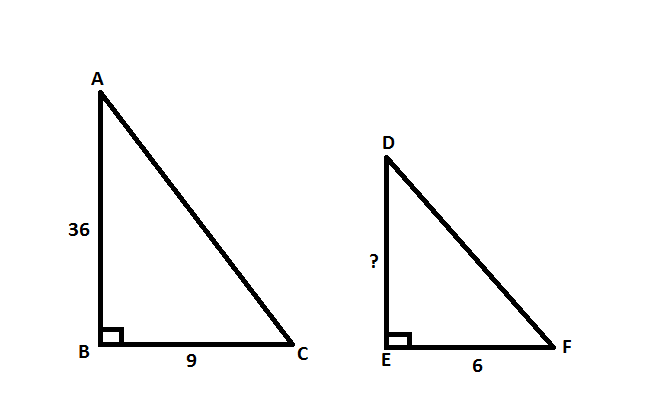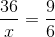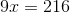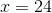## Example Questions

### Example Question #1 : Right Triangles

You have two right triangles that are similar.  The base of the first is 6 and the height is 9.  If the base of the second triangle is 20, what is the height of the second triangle?

23

35

33

25

30

30

Explanation:

Similar triangles are proportional.

Base1 / Height1 = Base2 / Height2

6 / 9 = 20 / Height2

Cross multiply  and solve for Height2

6 / 9 = 20 / Height2

6 * Height2=  20 * 9

Height2=  30

### Example Question #3 : Right Triangles

A right triangle is defined by the points (1, 1), (1, 5), and (4, 1).  The triangle's sides are enlarged by a factor of 3 to form a new triangle.  What is the area of the new triangle?

81 square units

36 square units

54 square units

None of the answers are correct

108 square units

54 square units

Explanation:

The points define a 3-4-5 right triangle.  Its area is A = 1/2bh = ½(3)(4) = 6.  The scale factor (SF) of the new triangle is 3.  The area of the new triangle is given by Anew = (SF)2 x (Aold) =

32 x 6 = 9 x 6 = 54 square units (since the units are not given in the original problem).

NOTE:  For a volume problem:  Vnew = (SF)3 x (Vold).

### Example Question #143 : Act Math

On a flat street, a light pole 36 feet tall casts a shadow that is 9 feet long. At the same time of day, a nearby light pole casts a shadow that is 6 feet long. How many feet tall is the second light pole?Explanation:

Start by drawing out the light poles and their shadows.In this case, we end up with two similar triangles. We know that these are similar triangles because the question tells us that these poles are on a flat surface, meaning angle B and angle E are both right angles. Then, because the question states that the shadow cast by both poles are at the same time of day, we know that angles C and F are equivalent. As a result, angles A and D must also be equivalent.

Since these are similar triangles, we can set up proportions for the corresponding sides.Now, solve forby cross-multiplying.